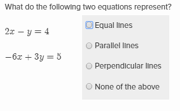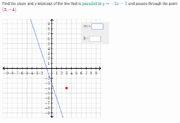Equations of parallel and perpendicular lines
Description
Exercise Name: Equations of parallel and perpendicular lines
Math Missions: High school geometry Math Mission
Types of Problems: 2

The Equations of parallel and perpendicular lines exercise appears under the High school geometry Math Mission. This exercise reinforces recognition of parallel and perpendicular lines.

## Types of Problems

There are two types of problems in this exercise:

1. Tell what the lines represent: This problem provides two equations that could be perpendicular, parallel, the same line, or none of the above. The user is asked to select which option is correct.Tell what the lines represent
2. Find equation of appropriate line: This problem provides a line and a point. Users are expected to find the equation of a line that is perpendicular or parallel to the give line and though the given point.Find equation of appropriate line

## Strategies

Knowledge of lines and comfort with slope are critical to being able to perform this exercise accurately and efficiently.

1. The line that is requested is going to be in slope-intercept form.
2. Slopes can be used to determine whether lines are parallel or perpendicular. Parallel lines have equal slopes and perpendicular lines have slopes that are negative reciprocals.
3. Sometimes it is possible to use the coefficients to find ratios and determine if lines are parallel or the actual same line.

## Real-life Applications

1. Lines have applications in business in the form of supply and demand curves, or cost, revenue and profit functions.
2. The ancient Egyptians from over 4000 years ago were very good at shapes and geometry. Every time the Nile burst its banks and the planes, they had to use geometry to measure their gardens and fields all over again.